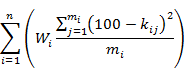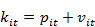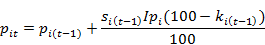# Study Plan Generator

Jonathan Searcy, March 2017

## Introduction

I first wrote this study plan generator in C++ to help my son plan for his GCSE examinations in 2017. I later converted it to HTML-5 and Javascript to make it more accessible. At the end of 2017 it was used as the program to be tested in the 2017 E-Genting Bug Hunt.

It is a Monte Carlo optimizer that comes up with a random study plan and then makes a random change to the study plan and if the change results in a better outcome, it is adopted, otherwise it is discarded. Another random change is then attempted until the maximum number of cycles is achieved at which time the results are displayed.

## Input Parameters

The study plan generator receives the following parameters:

1. start date;
2. number of study periods per day (1 to 12);
3. number of Monte Carlo cycles to execute;
4. for each subject:
1. subject code;
2. base permanent knowledge (0 to 100%);
3. base volatile knowledge (0 to 100%);
4. incremental permanent knowledge (0 to 100%);
5. incremental volatile knowledge (0 to 100%);
6. volatile knowledge half-life (1 to 999 days);
7. subject weight;
8. for each exam in the subject:
1. exam date;
2. exam period number.

The start date is the date of the first day in the study schedule. It is the date of the first day on which you are available to start studying.

Each day is divided into a number of study periods. Typically this would be 3 for morning, afternoon and evening, but it may be more or less depending on the exam schedule and your needs.

The number of Monte Carlo cycles is simply the number of experiments that the model makes in its effort to search for an optimal study plan. At least in theory, the larger the number of Monte Carlo cycles, the more likely it is that the study plan is closer to the optimal study plan.

The subject code is a code that identifies the subject to be studied during a study period.

Your knowledge is divided into permanent knowledge and volatile knowledge. Your permanent knowledge is that component of your knowledge that is not lost as a consequence of the elapse of time whereas volatile knowledge is expected to be lost in an exponential decay as time elapses.

Your knowledge is further divided into base knowledge and incremental knowledge. Your base knowledge is the knowledge that you possesses at the beginning of the study plan whereas incremental knowledge is the knowledge that your acquire during the study plan.

Your base permanent and volatile knowledge are the percentages of the subject material that you possesses at the beginning of the study plan. The permanent component is not lost over time whereas the volatile component is lost over time. For example, if you fully understand half the material at the beginning of the study plan, but a fifth of that knowledge was volatile and likely to be forgotten, your base permanent knowledge would be 40% and his base volatile knowledge would be 10%.

Your incremental permanent and volatile knowledge are the percentages of the remaining subject material that you are able to learn in a study period divided into permanent and volatile components. For example, if you are able to learn a fifth of the remaining material in a study period, but half of that is likely to be lost over time, your incremental permanent and volatile knowledge percentages would both be 10%.

The volatile knowledge half-life is the number of days it takes for half of your volatile knowledge to be lost. For example, if your base permanent and volatile knowledge were 40% and 10% respectively and your volatile knowledge half-life was 14 days and you did not study at all, then after 14 days your permanent and volatile knowledge percentages would have diminished to 40% and 5% respectively giving a total of 45%.

The subject weight is a means for giving more importance to certain subjects. For example, if your want to continue to further studies in engineering then mathematics, physics and chemistry might be more important than literature and history, whereas if you intend to study law, the opposite might be true. Subject weights are relative. Conceptually, a subject with a weight of 2 is twice as important as a subject with a weight of 1.

The exam dates are the dates on which the exams in the subject are scheduled. The exam period numbers are the period in the day in which the exam is to occur. Period numbers start at zero for the first period of the day.

## Saving and Restoring Parameters

The study plan generator has a means to save a configuration of entered parameters in nonvolatile storage identified by a file name and having saved the parameters, has a means to restore the saved parameters back into the data entry fields so that they can be used again without having to enter all the values.

## Objective Equation

The study plan generator experiments with assigning different subjects to different study periods and then selecting the combination that minimises the value of the following equation (the 'Objective Equation'):Where: i is the subject index. n is the total number of subjects. j is the exam index. mi is the number of exams in the i-th subject. Wi is the weight of the i-th subject. kij is the percentage of the material in the i-th subject that you possess at the time of the j-th exam in the subject.## Search Algorithm

The study plan generator displays a button entitled ‘Run’ that initiates a search for a combination of subjects to study in the available study periods that minimises the value of the Objective Equation.

The search proceeds as follows:

1. generate an initial ‘best study timetable’:
1. pseudo-randomly assign subjects to the available study periods;
2. calculate the value of the Objective Equation;
2. for the number of Monte Carlo cycles specified in the parameters less one:
1. copy the best study timetable to an experimental study timetable;
2. for each available study period:
1. select a pseudo-random value;
2. if that pseudo-random value is less than 3% of its range:
1. change the subject to be studied to a pseudo-randomly selected subject;
3. calculate the value of the Objective Equation for the experimental study timetable;
4. if the value of the Objective Equation for the experimental study timetable is less than that of the best study timetable:
1. copy the experimental study timetable to the best study timetable.

The study plan generator does not attempt to assign a subject to a study period if an exam is scheduled to take place during that study period. In the above pseudo code, an ‘available study period’ is a period during which no exam is scheduled.

The study plan generator does not attempt to assign a subject to a study period if the study period is after the last exam in that subject.

When all the cycles have been completed, the best study timetable is the most optimal study timetable that the algorithm can discover.

## Output

When the search algorithm described in the previous section finishes, the study plan generator displays the following information:

1. for each day in the best study timetable:
1. date;
2. for each study period in the best study timetable:
1. if the study period is occupied by an exam:
1. subject code of the exam;
2. the character ‘*’ to flag the exam;
3. forecast result of the exam;
2. else:
1. subject code of the subject to be studied during the period;
2. value of the objective equation for the best study timetable.